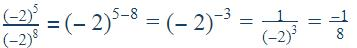Courses

# Test: Exponents And Powers- 1

## 10 Questions MCQ Test Mathematics (Maths) Class 8 | Test: Exponents And Powers- 1

Description
This mock test of Test: Exponents And Powers- 1 for Class 8 helps you for every Class 8 entrance exam. This contains 10 Multiple Choice Questions for Class 8 Test: Exponents And Powers- 1 (mcq) to study with solutions a complete question bank. The solved questions answers in this Test: Exponents And Powers- 1 quiz give you a good mix of easy questions and tough questions. Class 8 students definitely take this Test: Exponents And Powers- 1 exercise for a better result in the exam. You can find other Test: Exponents And Powers- 1 extra questions, long questions & short questions for Class 8 on EduRev as well by searching above.
QUESTION: 1

### (−2)5÷ (−2)8 = ?

Solution:QUESTION: 2

### Write the expression using exponents: 61 × 61 × 61 × 61 × 61

Solution:

Answers is  61^5 because 61 is multiplied 5 times

QUESTION: 3

### Find the value of x, if 32 = 2x.

Solution:

Factorisation of 32 is 2 x 2 x 2 x 2 x 2
32 = 25
X = 5

QUESTION: 4

The repeated factor in an exponential expression is called ____.

Solution:

An expression that represents repeated multiplication of the same factor is called a power. The exponent corresponds to the number of times the base is used as a factor.

QUESTION: 5

A group of students were given an assignment to collect different types of leaves. The group collected32 types of leaves. Represent the number of leaves collected in the form of exponential expression with its base being indivisible.

Solution:

25 = 2 x 2 x 2 x 2 x 2

2 x 2 = 4

4 x 2 = 8

8 x 2 = 16

16 x 2 = 32

QUESTION: 6

Find the value of the expression a2 for a = 10.

Solution:

Multiply 10 twice i.e 10 × 10 because they asked 'a' to the power 2 . so multiply by twice, the solution is 100 .

QUESTION: 7

Evaluate the exponential expression (−b)× (−b)5, for b = 4.

Solution:

(-b)4= (-44) =(-4 × -4) × (-4 × -4)

=16×16 (as -×-=+) =256

(-b)5=1024

so, -1024 × 256 = -262144

QUESTION: 8

When we have to add numbers in standard form, we convert them into numbers with the ________ exponents.

Solution:

For example , 2.38 x 10+ 21.1 x 10= 2.38 x 10+ 2.11 x 10= 4.49 x 104

QUESTION: 9

Simplify and write in exponential form: (−2)−3 × (−2)− 4

Solution:

(-2)-3*(-2)-4 = (-2)(-3)+(-4)  = (-2)(-7)

QUESTION: 10

Evaluate: 82

Solution:

8= 8 x 8 = 64